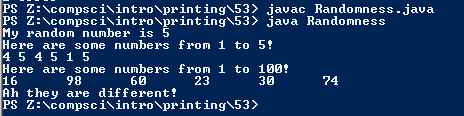# Randomness

## Code

```    /// Name: Mahima Choudhary
/// Period: 6
/// Program Name: Randomness
/// File Name: Randomness.java
/// Date Finished: 11/30/2015

import java.util.Random;

public class Randomness {

public static void main( String[] args ){

Random r = new Random();
/// seed numbers cause for a result of the same number everytime.

int x = 1 + r.nextInt(10);

System.out.println( "My random number is " + x );

System.out.println( "Here are some numbers from 1 to 5!" );
System.out.print( 2 + r.nextInt(5) + " " );
System.out.print( 2 + r.nextInt(5) + " " );
System.out.print( 2 + r.nextInt(5) + " " );
System.out.print( 2 + r.nextInt(5) + " " );
System.out.print( 2 + r.nextInt(5) + " " );
System.out.print( 2 + r.nextInt(5) + " " );
System.out.println();
/// The numbers are in the range 0-4 when the 1+ is deleted.
/// The numbers are in the range 3-7 when the 3+ is added.

System.out.println( "Here are some numbers from 1 to 100!" );
System.out.print( 4 + r.nextInt(100) + "\t" );
System.out.print( 4 + r.nextInt(100) + "\t" );
System.out.print( 4 + r.nextInt(100) + "\t" );
System.out.print( 4 + r.nextInt(100) + "\t" );
System.out.print( 4 + r.nextInt(100) + "\t" );
System.out.print( 4 + r.nextInt(100) + "\t" );
System.out.println();

int num1 = 1 + r.nextInt(10);
int num2 = 1 + r.nextInt(10);

if ( num1 == num2 ) {
System.out.println( "Did you notice they are the same?." );
}

if ( num1 != num2 ) {
System.out.println( "Ah they are different!" );
}

}
}    /// Name: Mahima Choudhary
/// Period: 6
/// Program Name: Randomness
/// File Name: Randomness.java
/// Date Finished: 11/30/2015

import java.util.Random;

public class Randomness {

public static void main( String[] args ){

Random r = new Random();
/// seed numbers cause for a result of the same number everytime.

int x = 1 + r.nextInt(10);

System.out.println( "My random number is " + x );

System.out.println( "Here are some numbers from 1 to 5!" );
System.out.print( 2 + r.nextInt(5) + " " );
System.out.print( 2 + r.nextInt(5) + " " );
System.out.print( 2 + r.nextInt(5) + " " );
System.out.print( 2 + r.nextInt(5) + " " );
System.out.print( 2 + r.nextInt(5) + " " );
System.out.print( 2 + r.nextInt(5) + " " );
System.out.println();
/// The numbers are in the range 0-4 when the 1+ is deleted.
/// The numbers are in the range 3-7 when the 3+ is added.

System.out.println( "Here are some numbers from 1 to 100!" );
System.out.print( 4 + r.nextInt(100) + "\t" );
System.out.print( 4 + r.nextInt(100) + "\t" );
System.out.print( 4 + r.nextInt(100) + "\t" );
System.out.print( 4 + r.nextInt(100) + "\t" );
System.out.print( 4 + r.nextInt(100) + "\t" );
System.out.print( 4 + r.nextInt(100) + "\t" );
System.out.println();

int num1 = 1 + r.nextInt(10);
int num2 = 1 + r.nextInt(10);

if ( num1 == num2 ) {
System.out.println( "Did you notice they are the same?." );
}

if ( num1 != num2 ) {
System.out.println( "Ah they are different!" );
}

}
}
```

### Picture of the output